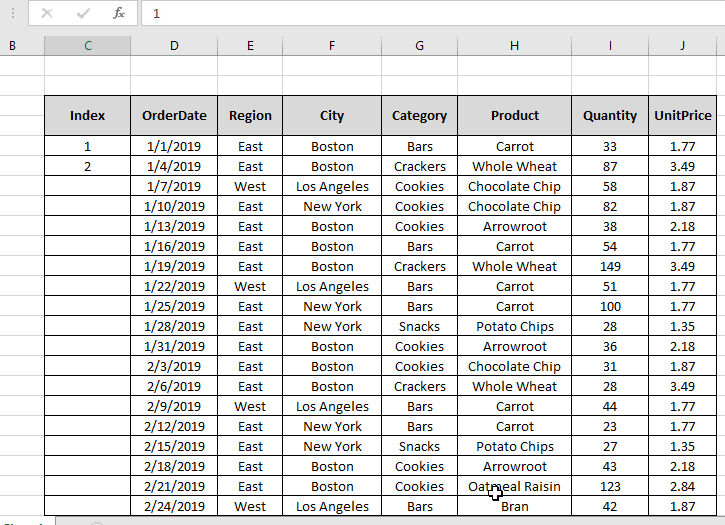# Get Relative ROW index in excel

For instance, we have a data and we need to mark the index for the data having index column. But the problem is that the data doesn't start from the 1st row of the worksheet. So we will use the ROW function and a simple logic to get the Index for the data. Let's get some understanding for the ROW function first.

The ROW function returns the row number of the cell or the cell reference. It returns a number for the given cell reference.

Syntax:

 =ROW([cell_reference])

Note: If no argument is given to the function. Then the function returns the row number for the cell where formula is used.

Now we will construct a formula using the above explained function and a simple logic. Firstly, we need to get the first index which is 1. So we need to create a difference of 1 between 2 numbers. These two numbers will be the outcome of two ROW functions. Then for the rest of the table we just be extending the same formula till the last row of the data.

Use the formula:

 = ROW() - ROW(1 - Row())

Explanation:

• ROW() function returns the row number for the cell where used.
• Now we want difference of 1. So we take 1 less number using the ROW(cell_reference) for the just previous cell.
• The difference will automatically create index

Example:

Let’s understand this function using it in an example.

Here we have a data_table with an INDEX column with it.We need to fill the INDEX column using the below formula

Use the formula in the C7 cell:

 = ROW( ) - ROW ( \$C\$6 )

Explanation:

• ROW ( ) will return 7, the corresponding row number for the cell.
• ROW( \$C\$6 ) will return 6, the row number for the C^ cell.
• \$ sign with cell reference is used to fix the cell reference as excel extends the cell reference as you extend the use of the formula.As you can see in the above snapshot that the formula returns 1 as the first INDEX for the table. Copy the formula to the rest of the cells using the Ctrl + D shortcut key or drag down option in excel.As you can see from the above snapshot that the index column is filled with values as required.

There's a new and easy method to the same. In Excel 2016, it automatically extends the values just by the drag down option. Follow the steps to see how it is used.

1. Type the number 1 & 2 in the first and second Index space.
2. Then select the two cells and drag it down till the Index column fills.As you can see in the gif above that the INDEX column is done.

Hope you understood how to Get Relative ROW index in Excel. Explore more articles on Excel cell reference function here. Please feel free to state your query or feedback for the above article.

Related Articles

How to use the ROW function in Excel

How to use the ROWS function in Excel

How to Remove Text in Excel Starting From a Position

Validation of text entries

Create drop down list in excel with colour

Remove leading and trailing spaces from text in Excel

Popular Articles

Edit a dropdown list

If with conditional formatting

If with wildcards

Vlookup by date

Terms and Conditions of use

The applications/code on this site are distributed as is and without warranties or liability. In no event shall the owner of the copyrights, or the authors of the applications/code be liable for any loss of profit, any problems or any damage resulting from the use or evaluation of the applications/code.